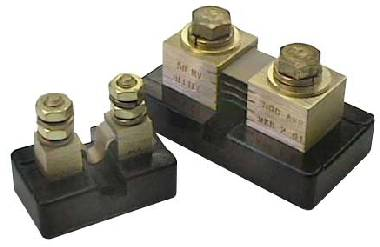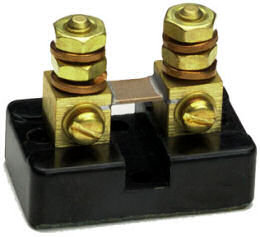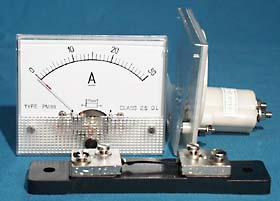# What is a ShuntA shunt (aka a current shunt resistor or an ammeter shunt) is a high precision resistor which can be used to measure the current flowing through a circuit. Using Ohm’s Law we know that the voltage dropped across a resistor divided by the resistance of that resistor is equal to the current, therefore if we measure the voltage across a shunt resistor in a circuit, we can easily calculate the current.For example, pictured above is a typical 100 Amp Shunt Resistor. This can be used to measure currents of up to 100 Amps – although to prevent overheating it should really only be used to measure continuous currents of no more than 60-70 Amps.
* If a shunt resistor overheats it can permanently change the resistance of the shunt.

This shunt is calibrated such that the voltage drop across it is 100mV when the current flowing through it is 100 Amps. Therefore we can calculate the resistance of this shunt to be voltage divided by current = 0.1 / 100 = 0.001 Ohms (typically to within ± 0.25% accuracy). Therefore if a voltage drop of 28mV is measured (using a standard multimeter or 0-100mV range voltmeter), we know that the current flowing is 0.028/0.001 = 28 Amps.
(The power wasted by the shunt resistor is given by multiplying the voltage by the current = 0.028 * 28 = 0.78 Watts in this example.)

To save making this calculation manually each time it is possible to re-label a 0-100mV moving coil voltmeter so it instead reads 0-100 Amps. This would be achieved simply by sticking the word “Amps” over “mV” on the face of the meter which would now be an ammeter rather than a voltmeter.### Using a Shunt Resistor in a Renewable Energy System

It is very important to know how much current is flowing in and out of the battery bank in a renewable energy system. When charging the current flowing into the batteries should never be more than 10% of the battery capacity – e.g. a 100Ah battery should not be charged with more than a 10 Amp current or it may be damaged and/or overheat.

It is also very useful to know how much current is being generated by a wind turbine or solar panel, because that information helps you to calculate how much power is being generated. For example a 12 Volt 15 Watt PV Solar Panel may produce a voltage of 18 Volts when it is very cloudy and 21 Volts when it is very sunny.
When it is cloudy you may measure a current of just 0.1 Amps and when it is sunny a current of 0.8 Amps. Power is equal to voltage multiplied by current, so the solar panel is generating just 2 Watts when it is cloudy and almost 17 Watts when it is sunny.

### Make Your Own Shunt Resistors

It is possible to Make a Basic Shunt Resistor very cheaply with some copper wire. Click here to find out more.